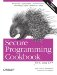# Obtaining the Current Date and Time

Problem

You want to retrieve the current date and time from the user's computer, either as a local time or as a Coordinated Universal Time (UTC).

Solution

Call the time function from the header, passing a value of 0 as the parameter. The result will be a time_t value. You can use the gmtime function to convert the time_t value to a tm structure representing the current UTC time (a.k.a. Greenwich Mean Time or GMT); or, you can use the localtime function to convert the time_t value to a tm structure representing the local time. The program in Example 5-1 obtains the current date/time, and then converts it to local time and outputs it. Next, the program converts the current date/time to a UTC date/time and outputs that.

Example 5-1. Getting the local and UTC times

```#include
#include
#include

using namespace std;

int main( )
{
// Current date/time based on current system
time_t now = time(0);

// Convert now to tm struct for local timezone
tm* localtm = localtime(&now);
cout << "The local date and time is: " << asctime(localtm) << endl;

// Convert now to tm struct for UTC
tm* gmtm = gmtime(&now);
if (gmtm != NULL) {
cout << "The UTC date and time is: " << asctime(gmtm) << endl;
}
else {
cerr << "Failed to get the UTC date and time" << endl;
return EXIT_FAILURE;
}
}```

Discussion

The time function returns a time_t type, which is an implementation-defined arithmetic type for representing a time period (a.k.a. a time interval) with at least a resolution of one second. The largest time interval that can be portably represented using a time_t is 2,147,483,648 seconds, or approximately 68 years.

A call to time(0) returns a time_t representing the time interval from an implementation defined base time (commonly 0:00:00 January 1, 1970) to the current moment.

## The Year 2038 Bug

Since a time_t is only required to represent time intervals of 68 years, and many implementations use a base year of 1970 for representing the current time, there is an inability for many popular C++ implementations to represent dates and times after 2038. This means that a lot of software could break in 2038, if programmers don't take adequate precautions.

A more workable representation of the current date and time is achieved by converting to a tm struct using the localtime or gmtime functions. A tm struct has the integer fields shown in Example 5-2.

Example 5-2. Layout of a tm struct

```struct tm {
int tm_sec; // seconds of minutes from 0 to 61 (60 and 61 are leap seconds)
int tm_min; // minutes of hour from 0 to 59
int tm_hour; // hours of day from 0 to 24
int tm_mday; // day of month from 0 to 23
int tm_mon; // month of year from 0 to 11
int tm_year; // year since 1900
int tm_wday; // days since sunday
int tm_yday; // days since January 1st
int tm_isdst; // hours of daylight savings time
}```

When using the gmtime function, be sure to check its return value. If the computer running the code doesn't have a local time zone defined, the gmtime function will be unable to compute the UTC time, and will return 0. If you pass 0 to the asctime function, undefined behavior will result.

The localtime, gmtime, and asctime functions all return pointers to statically allocated objects. This is more efficient for the library, but it means that subsequent calls will change the value of those objects. The code in Example 5-3 shows how this can have surprising effects.

Example 5-3. Pitfalls of using asctime

```void f( ) {
char* x = asctime(localtime(time(0)));
wait_for_15_seconds( ); // do some long processing task
asctime(localtime(time(0)));
cout << x << endl; // prints out the current time, not fifteen seconds ago

.
}```Secure Programming Cookbook for C and C++: Recipes for Cryptography, Authentication, Input Validation & More
ISBN: 0596003943
EAN: 2147483647
Year: 2006
Pages: 241